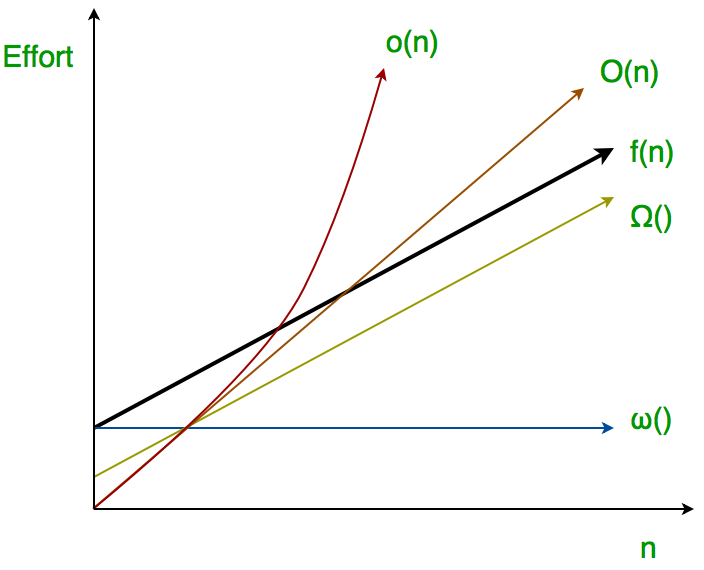# Analysis of algorithms | little o and little omega notations

• Difficulty Level : Easy
• Last Updated : 19 Apr, 2020

The main idea of asymptotic analysis is to have a measure of efficiency of algorithms that doesn’t depend on machine specific constants, mainly because this analysis doesn’t require algorithms to be implemented and time taken by programs to be compared. We have already discussed Three main asymptotic notations. The following 2 more asymptotic notations are used to represent time complexity of algorithms.

Little ο asymptotic notation

Attention reader! Don’t stop learning now. Get hold of all the important DSA concepts with the DSA Self Paced Course at a student-friendly price and become industry ready.  To complete your preparation from learning a language to DS Algo and many more,  please refer Complete Interview Preparation Course.

In case you wish to attend live classes with experts, please refer DSA Live Classes for Working Professionals and Competitive Programming Live for Students.

Big-Ο is used as a tight upper-bound on the growth of an algorithm’s effort (this effort is described by the function f(n)), even though, as written, it can also be a loose upper-bound. “Little-ο” (ο()) notation is used to describe an upper-bound that cannot be tight.

Definition : Let f(n) and g(n) be functions that map positive integers to positive real numbers. We say that f(n) is ο(g(n)) (or f(n) Ε ο(g(n))) if for any real constant c > 0, there exists an integer constant n0 ≥ 1 such that 0 ≤ f(n) < c*g(n).Thus, little o() means loose upper-bound of f(n). Little o is a rough estimate of the maximum order of growth whereas Big-Ο may be the actual order of growth.

In mathematical relation,
f(n) = o(g(n)) means
lim  f(n)/g(n) = 0
n→∞

Examples:

Is 7n + 8 ∈ o(n2)?
In order for that to be true, for any c, we have to be able to find an n0 that makes
f(n) < c * g(n) asymptotically true.
lets took some example,
If c = 100,we check the inequality is clearly true. If c = 1/100 , we’ll have to use
a little more imagination, but we’ll be able to find an n0. (Try n0 = 1000.) From
these examples, the conjecture appears to be correct.
then check limits,
lim  f(n)/g(n) = lim  (7n + 8)/(n2) = lim  7/2n = 0 (l’hospital)
n→∞ n→∞ n→∞

hence 7n + 8 ∈ o(n2)

Little ω asymptotic notation

Definition : Let f(n) and g(n) be functions that map positive integers to positive real numbers. We say that f(n) is ω(g(n)) (or f(n) ∈ ω(g(n))) if for any real constant c > 0, there exists an integer constant n0 ≥ 1 such that f(n) > c * g(n) ≥ 0 for every integer n ≥ n0.

f(n) has a higher growth rate than g(n) so main difference between Big Omega (Ω) and little omega (ω) lies in their definitions.In the case of Big Omega f(n)=Ω(g(n)) and the bound is 0<=cg(n)<=f(n), but in case of little omega, it is true for 0<=c*g(n)<f(n).

The relationship between Big Omega (Ω) and Little Omega (ω) is similar to that of Big-Ο and Little o except that now we are looking at the lower bounds. Little Omega (ω) is a rough estimate of the order of the growth whereas Big Omega (Ω) may represent exact order of growth. We use ω notation to denote a lower bound that is not asymptotically tight.
And, f(n) ∈ ω(g(n)) if and only if g(n) ∈ ο((f(n)).

In mathematical relation,
if f(n) ∈ ω(g(n)) then,

lim  f(n)/g(n) = ∞
n→∞

Example:
Prove that 4n + 6 ∈ ω(1);

the little omega(ο) running time can be proven by applying limit formula given below.
if lim  f(n)/g(n) = ∞ then functions f(n) is ω(g(n))
n→∞
here,we have functions f(n)=4n+6 and g(n)=1
lim   (4n+6)/(1) = ∞
n→∞
and,also for any c we can get n0 for this inequality 0 <= c*g(n) < f(n), 0 <= c*1 < 4n+6
Hence proved.